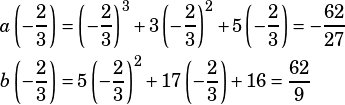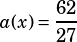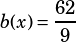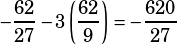Would you please show an easy way to solve this:

a(x) = x^3 +3x^2 + 5x
b(x) = 5x^2 + 17x + 16

Polynomial a(x) and b(x) are defined above. Which of the polynomials below has a factor of 3x + 2 ?

(A) l(x) = a(x)+ b(x)
(B) m(x) = 3a(x) + b(x)
(C) n(x) = a(x) – 3b(x)
(D) p(x) = 2a(x) + 3b(x)

This is a ripoff of Official Test 4 Section 4 #25, but it’s a bad ripoff because the original question could be easily factored for a clever and quick solution, and this one can’t. If you get a question like this, you’ll be allowed to use your calculator, though, so the following grind-it-out solution shouldn’t actually take that long.

Note that if a polynomial has a factor of 3x + 2, then it will also have a zero at. So the cleverest thing I can think of to do is calculateand, and then plug those into each answer choice until one gives you zero. Like so:Now try each answer choice to see which equals zero whenand. You might be able to see it without much trouble…

A)B)C)D)Another way to go is to graph each choice with your calculator and look for a zero at. That feels to me like it’ll be a bit time consuming, but if you just put your head down and started working on that the minute you saw this question, I bet you’d be done with it within 90 seconds, which is a fine amount of time to spend on a hard question in the calculator section.